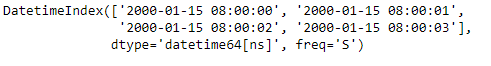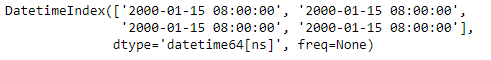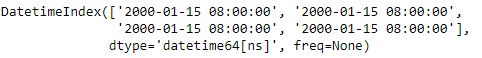Related Articles
Python | Pandas DatetimeIndex.floor()
• Last Updated : 29 Dec, 2018

Python is a great language for doing data analysis, primarily because of the fantastic ecosystem of data-centric python packages. Pandas is one of those packages and makes importing and analyzing data much easier.

Pandas` DatetimeIndex.floor()` function floor the data to the specified frequency. The function takes the target frequency as input. It returns a new DatetimeIndex object.

Syntax: DatetimeIndex.floor(freq)

Parameters :
freq : The frequency level to floor the index to. Must be a fixed frequency like ‘S’ (second) not ‘ME’ (month end).

Return : Index of the same type for a DatetimeIndex or TimedeltaIndex, or a Series with the same index for a Series.

Example #1: Use `DatetimeIndex.floor()` function to floor the data of the DatetimeIndex object to the specified frequency.

 `# importing pandas as pd``import` `pandas as pd`` ` `# Create the DatetimeIndex``# Here 'S' represents secondly frequency ``didx ``=` `pd.DatetimeIndex(start ``=``'2000-01-15 08:00'``, freq ``=``'S'``, periods ``=` `4``)`` ` `# Print the DatetimeIndex``print``(didx)`

Output :Now we want to floor the second based frequency of the DatetimeIndex object to minute based frequency

 `# convert to the passed frequency``# 'T' represents minute based frequency``didx.floor(``'T'``)`

Output :As we can see in the output, the function has floored the values of the DatetimeIndex object to the desired frequency.

Example #2: Use `DatetimeIndex.floor()` function to floor the data of the DatetimeIndex object to the specified frequency.

 `# importing pandas as pd``import` `pandas as pd`` ` `# Create the DatetimeIndex``# Here 'T' represents minutely frequency ``didx ``=` `pd.DatetimeIndex(start ``=``'2000-01-15 08:00'``, freq ``=``'T'``, periods ``=` `4``)`` ` `# Print the DatetimeIndex``print``(didx)`

Output :Now we want to floor the minute based frequency of the DatetimeIndex object to hour based frequency

 `# floor minute based frequency to hour based frequency``didx.floor(``'H'``)`

Output :As we can see in the output, the function has floored the values of the DatetimeIndex object to the desired frequency.

Attention geek! Strengthen your foundations with the Python Programming Foundation Course and learn the basics.

To begin with, your interview preparations Enhance your Data Structures concepts with the Python DS Course. And to begin with your Machine Learning Journey, join the Machine Learning – Basic Level Course

My Personal Notes arrow_drop_up